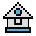Home

# OCT\$ Function

#### Purpose:

To convert a decimal value to an octal value.

#### Syntax:

`OCT\$(x)`

x is rounded to an integer before OCT\$(x) is evaluated.

This statement converts a decimal value within the range of -32768 to +65535 to an octal string expression.

Octal numbers are numbers to the base 8 rather than base 10 (decimal numbers).

See the HEX\$ function for hexadecimal conversion.

#### Examples:

```10 PRINT OCT\$(18)
RUN
Ā22```

Decimal 18 equals octal 22.